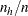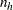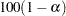STRATA Statement

STRATA variables </ options> ;

The STRATA statement names variables that partition the input data set into nonoverlapping subgroups (strata). The combinations of levels of STRATA variables define the strata. PROC SURVEYSELECT then selects independent samples from these strata, according to the selection method and design parameters that you specify in the PROC SURVEYSELECT statement. For information about the use of stratification in sample design, see Lohr (2010), Kalton (1983), Kish (1965, 1987), and Cochran (1977).

The STRATA variables are one or more variables in the DATA= input data set. These variables can be either character or numeric, but the procedure treats them as categorical variables. The formatted values of the STRATA variables determine the STRATA variable levels. Thus, you can use formats to group values into levels. See the discussion of the FORMAT procedure in the Base SAS Procedures Guide and the discussions of the FORMAT statement and SAS formats in SAS Language Reference: Dictionary.

The STRATA variables function much like BY variables, and PROC SURVEYSELECT expects the input data set to be sorted in order of the STRATA variables.

If you specify a CONTROL statement, or if you specify METHOD=PPS, the input data set must be sorted in ascending order by the STRATA variables. This means you cannot use the STRATA option NOTSORTED or DESCENDING when you specify a CONTROL statement or METHOD=PPS.

If your input data set is not sorted by the STRATA variables in ascending order, use one of the following alternatives:

• Sort the data by using the SORT procedure with the STRATA variables in a BY statement.

• Specify the NOTSORTED or DESCENDING option in the STRATA statement (when you do not specify a CONTROL statement or METHOD=PPS). The NOTSORTED option does not mean that the data are unsorted but rather that the data are arranged in groups (according to values of the STRATA variables) and that these groups are not necessarily in alphabetical or increasing numeric order.

• Create an index on the STRATA variables by using the DATASETS procedure (in Base SAS software).

### Allocation Options

The STRATA options request allocation of the total sample size among the strata. You can use the ALLOC= option to specify the allocation method. Available allocation methods include proportional allocation (ALLOC=PROP), optimal allocation (ALLOC=OPTIMAL), and Neyman allocation (ALLOC=NEYMAN). See the section Sample Size Allocation for details about these methods.

Instead of requesting that PROC SURVEYSELECT compute the sample allocation, you can provide the allocation proportions by using the ALLOC=(values) option or the ALLOC=SAS-data-set option. Then PROC SURVEYSELECT allocates the total sample size among the strata according to the proportions that you provide. Allocation proportions are relative stratum sample sizes,, whereis the stratumsample size andis the total sample size.

You can use the SAMPSIZE= option in the PROC SURVEYSELECT statement to specify the total sample size to be allocated among the strata. Alternatively, you can specify the desired margin of error in the MARGIN= option, and the procedure determines the stratum sample sizes that are required to achieve that margin. See the section Specifying the Margin of Error for details.

When you request sample allocation, by default PROC SURVEYSELECT computes the allocation of the total sample size among the strata and then selects the sample. If you specify the NOSAMPLE option, the procedure computes the allocation but does not select the sample. In this case the OUT= output data set contains the stratum sample sizes that are computed according to the specified allocation method. See the section Allocation Output Data Set for details.

You can use the ALLOC= option with any selection method except METHOD=PPS_BREWER and METHOD=PPS_MURTHY, which select two units from each stratum.

Table 91.2 summarizes the options available in the STRATA statement. Descriptions of the options follow in alphabetical order.

Table 91.2 STRATA Statement Options for Sample Allocation

Options

Specify the allocation method

Provide allocation proportions

Specify the margin of error

Provide stratum costs and variances

Specify the minimum sample size per stratum

Allocate but do not select the sample

You can specify the following options in the STRATA statement after a slash (/):

ALLOC=name

specifies the method for allocating the total sample size among the strata. The following values for name are available:

PROPORTIONAL | PROP

requests proportional allocation, which allocates the total sample size in proportion to the stratum sizes, where the stratum size is the number of sampling units in the stratum. See the section Proportional Allocation for details.

OPTIMAL | OPT

requests optimal allocation, which allocates the total sample size among the strata in proportion to stratum sizes, stratum variances, and stratum costs. See the section Optimal Allocation for more information. If you specify ALLOC=OPTIMAL, you must provide the stratum variances with the VAR=(values), VAR=SAS-data-set, or VAR option. You must provide the stratum costs with the COST=(values), COST=SAS-data-set, or COST option.

NEYMAN

requests Neyman allocation, which allocates the total sample size among the strata in proportion to the stratum sizes and variances. See the section Neyman Allocation for more information. If you specify ALLOC=NEYMAN, you must provide the stratum variances with the VAR=(values), VAR=SAS-data-set, or VAR option.

ALLOC=(values)

lists stratum allocation proportions. You can separate values with blanks or commas.

Each allocation proportion specifies the percent of the total sample size to allocate to the corresponding stratum. The number of ALLOC= values must equal the number of strata in the input data set. The sum of the allocation proportions must equal 1.

Each allocation proportion must be a positive number. You can specify each value as a number between 0 and 1. Or you can specify a value in percentage form as a number between 1 and 100, and PROC SURVEYSELECT converts that number to a proportion. The procedure treats the value 1 as 100% instead of 1%.

List the allocation proportions in the order in which the strata appear in the input data set. If you use the ALLOC=(values) option, the input data set must be sorted by the STRATA variables in ascending order. You cannot use the DESCENDING or NOTSORTED option in the STRATA statement.

ALLOC=SAS-data-set

names a SAS data set that contains stratum allocation proportions. You provide the stratum allocation proportions in the ALLOC= data set variable _ALLOC_.

Each allocation proportion specifies the percent of the total sample size to allocate to the corresponding stratum. The sum of the allocation proportions must equal 1.

Each allocation proportion must be a positive number. You can specify the value as a number between 0 and 1. Or you can specify the value in percentage form as a number between 1 and 100, and PROC SURVEYSELECT converts that number to a proportion. The procedure treats the value 1 as 100% instead of 1%.

The ALLOC= data set should contain all the STRATA variables, with the same type and length as in the DATA= input data set. The STRATA groups should appear in the same order in the ALLOC= data set as in the DATA= data set. The ALLOC= data set is a secondary input data set. See the section Secondary Input Data Set for details. You can name only one secondary data set in each invocation of the procedure.

ALLOCMIN=n

specifies the minimum sample size to allocate to a stratum. When you specify ALLOCMIN=n, PROC SURVEYSELECT allocates at least n sampling units to each stratum. If you do not specify the ALLOCMIN= option, PROC SURVEYSELECT allocates at least one sampling unit to each stratum by default.

The minimum stratum sample size n must be a positive integer. The ALLOCMIN value n times the number of strata should not exceed the total sample size to be allocated. For without-replacement selection methods, the ALLOCMIN value should not exceed the number of sampling units in any stratum.

ALPHA=specifies the level of the confidence interval for the MARGIN= determination of stratum sample sizes. See the section Specifying the Margin of Error for details.

The value ofmust be between 0 and 1; the default is 0.05. A confidence level ofproduces a% confidence interval. The default of ALPHA=0.05 produces a 95% confidence interval.

COST

indicates that stratum costs are included in the secondary input data set. Use the COST option when you have already named the secondary input data set in another option, such as the VAR=SAS-data-set option. You provide the stratum costs in the secondary input data set variable _COST_.

A stratum cost represents the per-unit cost (the survey cost of a single unit in the stratum). Each stratum cost must be a positive number. Cost values are required if you specify the ALLOC=OPTIMAL option.

COST=(values)

specifies stratum costs, which are required if you specify the ALLOC=OPTIMAL option. You can separate values with blanks or commas.

A stratum cost represents the per-unit cost (the survey cost of a single unit in the stratum). Each stratum cost must be a positive number.

The number of COST= values must equal the number of strata in the input data set. List the stratum costs in the order in which the strata appear in the input data set. If you use the COST=values option, the input data set must be sorted by the STRATA variables in ascending order. You cannot use the DESCENDING or NOTSORTED option in the STRATA statement.

COST=SAS-data-set

names a SAS data set that contains the stratum costs. You provide the stratum costs in the COST= data set variable _COST_.

A stratum cost represents the per-unit cost (the survey cost of a single unit in the stratum). Each stratum cost must be a positive number. Stratum costs are required if you specify the ALLOC=OPTIMAL option.

The COST= data set should contain all the STRATA variables, with the same type and length as in the DATA= input data set. The STRATA groups should appear in the same order in the COST= data set as in the DATA= data set. The COST= data set is a secondary input data set. See the section Secondary Input Data Set for details. You can name only one secondary input data set in each invocation of the procedure.

MARGIN=value

specifies the desired margin of error for estimating the overall mean from the stratified sample. When you specify the MARGIN= option, PROC SURVEYSELECT determines the stratum sample sizes required to achieve this margin for the allocation method or proportions that you specify in the ALLOC= option. See the section Specifying the Margin of Error for details.

The MARGIN= value must be a positive number. When you specify the MARGIN= option, you must also provide the stratum variances in the VAR=(values), VAR=SAS-data-set, or VAR option.

You can use the ALPHA= option to set the level of the confidence interval that the MARGIN= computation uses. The default of ALPHA=0.05 specifies a 95% confidence interval.

You can request the MARGIN= option for any allocation method (proportional, optimal, or Neyman) or for allocation proportions that you provide (ALLOC=(values) or ALLOC=SAS-data-set). When you use the MARGIN= option, you cannot specify a total sample size in the SAMPSIZE= option in the PROC SURVEYSELECT statement.

NOSAMPLE

requests that PROC SURVEYSELECT allocate the total sample size among the strata but not select the sample. When you specify the NOSAMPLE option, the OUT= output data set contains the stratum sample sizes that PROC SURVEYSELECT computes. See the section Allocation Output Data Set for details.

STATS

displays statistics for the sample allocation. If you specify the MARGIN= option, the STATS option displays the expected margin of error for the allocation. See the section Specifying the Margin of Error for details. If you request ALLOC=OPTIMAL or ALLOC=NEYMAN without the MARGIN= option, the STATS option displays the expected variance, which is computed from the stratum variances that you provide and the allocated stratum sample sizes. If you request ALLOC=OPTIMAL, the STATS option also displays the total stratum cost, which is computed from the stratum costs that you provide and the allocated stratum sample sizes.

VAR

indicates that stratum variances are included in the secondary input data set. Use the VAR option when you have already named the secondary input data set in another option, such as the COST=SAS-data-set option. You provide the stratum variances in the secondary input data set variable _VAR_.

Each stratum variance must be a positive number. Stratum variances are required if you specify the ALLOC=OPTIMAL, ALLOC=NEYMAN, or MARGIN= option.

VAR=(values)

lists stratum variances, which are required if you specify the ALLOC=OPTIMAL, ALLOC=NEYMAN, or MARGIN= option. You can separate values with blanks or commas.

Each stratum variance must be a positive number. The number of VAR= values must equal the number of strata in the input data set. List the stratum variances in the order in which the strata appear in the input data set. If you use the VAR=(values) option, the input data set must be sorted by the STRATA variables in ascending order. You cannot use the DESCENDING or NOTSORTED option in the STRATA statement.

VAR=SAS-data-set

names a SAS data set that contains the stratum variances. You provide the stratum variances in the VAR= data set variable _VAR_.

Each stratum variance must be a positive number. Stratum variances are required if you specify the ALLOC=OPTIMAL, ALLOC=NEYMAN, or MARGIN= option.

The VAR= data set should contain all the STRATA variables, with the same type and length as in the DATA= input data set. The STRATA groups should appear in the same order in the VAR= data set as in the DATA= data set. The VAR= data set is a secondary input data set. See the section Secondary Input Data Set for details. You can name only one secondary input data set in each invocation of the procedure.